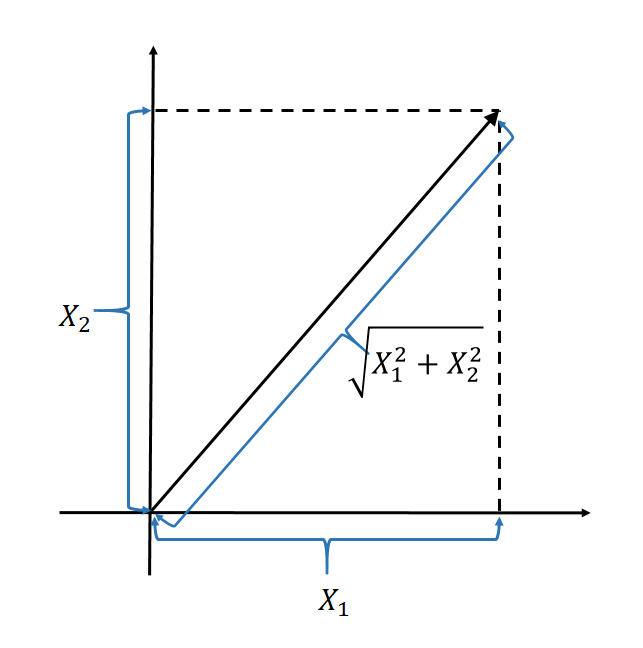13
Nov 16

## Statistical measures and their geometric roots

Variance, covariancestandard deviation and correlation: their definitions and properties are deeply rooted in the Euclidean geometry.

## Here is the why: analogy with Euclidean geometryEuclid axiomatically described the space we live in. What we have known about the geometry of this space since the ancient times has never failed us. Therefore, statistical definitions based on the Euclidean geometry are sure to work.

#### 1. Analogy between scalar product and covariance

Geometry. See Table 2 here for operations with vectors. The scalar product of two vectors$X=(X_1,...,X_n),\ Y=(Y_1,...,Y_n)$ is defined by$(X,Y)=\sum X_iY_i.$

Statistical analog: Covariance of two random variables is defined by$Cov(X,Y)=E(X-\bar{X})(Y-\bar{Y}).$

Both the scalar product and covariance are linear in one argument when the other argument is fixed.

#### 2. Analogy between orthogonality and uncorrelatedness

Geometry. Two vectors$X,Y$ are called orthogonal (or perpendicular) if

(1)$(X,Y)=\sum X_iY_i=0.$

Exercise. How do you draw on the plane the vectors$X=(1,0),\ Y=(0,1)$? Check that they are orthogonal.

Statistical analog: Two random variables are called uncorrelated if$Cov(X,Y)=0$.

#### 3. Measuring lengthsFigure 1. Length of a vector

Geometry: the length of a vector$X=(X_1,...,X_n)$ is$\sqrt{\sum X_i^2}$, see Figure 1.

Statistical analog: the standard deviation of a random variable$X$ is$\sigma(X)=\sqrt{Var(X)}=\sqrt{E(X-\bar{X})^2}.$

This explains the square root in the definition of the standard deviation.

#### 4. Cauchy-Schwarz inequality

Geometry$|(X,Y)|\le\sqrt{\sum X_i^2}\sqrt{\sum Y_i^2}$.

Statistical analog$|Cov(X,Y)|\le\sigma(X)\sigma(Y)$. See the proof here. The proof of its geometric counterpart is similar.

#### 5. Triangle inequalityFigure 2. Triangle inequality

Geometry$\sqrt{\sum (X_i+Y_i)^2}\le\sqrt{\sum X_i^2}+\sqrt{\sum X_i^2}$, see Figure 2 where the length of X+Y does not exceed the sum of lengths of X and Y.

Statistical analog: using the Cauchy-Schwarz inequality we have$\sigma(X+Y)=\sqrt{Var(X+Y)}$$=\sqrt{Var(X)+2Cov(X,Y)+Var(Y)}$$\le\sqrt{\sigma^2(X)+2\sigma(X)\sigma(Y)+\sigma^2(Y)}$$=\sigma(X)+\sigma(Y).$

#### 4. The Pythagorean theorem

Geometry: In a right triangle, the squared hypotenuse is equal to the sum of the squares of the two legs. The illustration is similar to Figure 2, except that the angle between X and Y should be right.

Proof. Taking two orthogonal vectors$X,Y$ as legs, we have

Squared hypotenuse =$\sum(X_i+Y_i)^2$

(squaring out and using orthogonality (1))$=\sum X_i^2+2\sum X_iY_i+\sum Y_i^2=\sum X_i^2+\sum Y_i^2$ = Sum of squared legs

Statistical analog: If two random variables are uncorrelated, then variance of their sum is a sum of variances$Var(X+Y)=Var(X)+Var(Y).$

#### 5. The most important analogy: measuring angles

Geometry: the cosine of the angle between two vectors$X,Y$ is defined by

Cosine between X,Y =$\frac{\sum X_iY_i}{\sqrt{\sum X_i^2\sum Y_i^2}}.$

Statistical analog: the correlation coefficient between two random variables is defined by$\rho(X,Y)=\frac{Cov(X,Y)}{\sqrt{Var(X)Var(Y)}}=\frac{Cov(X,Y)}{\sigma(X)\sigma(Y)}.$

This intuitively explains why the correlation coefficient takes values between -1 and +1.

Remark. My colleague Alisher Aldashev noticed that the correlation coefficient is the cosine of the angle between the deviations$X-EX$ and$Y-EY$ and not between$X,Y$ themselves.